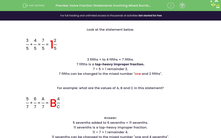# Solve Fraction Additions involving Mixed Numbers

In this worksheet, students will complete statements involving mixed numbers and improper fractions.Key stage:  KS 2

Curriculum topic:   Number: Fractions, Decimals and Percentages

Curriculum subtopic:   Convert Fractions

Difficulty level:#### Worksheet Overview

Look at the fraction sum below.

3/5 + 4/5 = 7/5

This means 3 fifths + 4 fifths = 7 fifths.

7 fifths is a top-heavy improper fraction.

7 ÷ 5 = remainder 2.

7 fifths can be changed to the mixed number one and 2 fifths.

7/5 = 1 2/5

5/7 + 6/7 = ?

5/7 + 6/7 = 11/7

5 sevenths added to 6 sevenths = 11 sevenths.

11 sevenths is a top-heavy improper fraction.

11 ÷ 7 = 1 remainder 4

11 sevenths can be changed to the mixed number one and 4 sevenths.

So, 11/7 = 1 4/7

Are you ready to have a go at some questions now?### What is EdPlace?

We're your National Curriculum aligned online education content provider helping each child succeed in English, maths and science from year 1 to GCSE. With an EdPlace account you’ll be able to track and measure progress, helping each child achieve their best. We build confidence and attainment by personalising each child’s learning at a level that suits them.

Get started